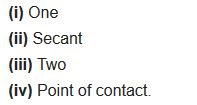# NCERT Solutions for Class 10 Maths Chapter 10 Circles Ex 10.1

Get Free NCERT Solutions for Class 10 Maths Chapter 10 Ex 10.1 PDF. Circles Class 10 Maths NCERT Solutions are extremely helpful while doing your homework. Exercise 10.1 Class 10 Maths NCERT Solutions were prepared by Experienced ncert-books.inTeachers. Detailed answers of all the questions in Chapter 10 Maths Class 10 Circles Exercise 10.1 provided in NCERT TextBook.

Question 1.
How many tangents can a circle have?
Solution:
There can be infinitely many tangents to a circle.

Question 2.
Fill in the blanks:
(i) A tangent to a circle intersects it in ………… point(s).
(ii) A line intersecting a circle in two points is called a ………… .
(iii) A circle can have ………………. parallel tangents at the most.
(iv) The common point of a tangent to a circle and the circle is called ……….. .
Solution:You can also download the free PDF of  Ex 10.1 Class 10 Circles NCERT Solutions or save the solution images and take the print out to keep it handy for your exam preparation.

Question 3.
A tangent PQ at a point P of a circle of radius 5 cm meets a line through the centre O at a point Q so that OQ = 12 cm. Length PQ is
(a) 12 cm
(b) 13 cm
(c) 8.5 cm
(d)$\sqrt{199}$cm
Solution:Question 4.
Draw a circle and two lines parallel to a given line such that one is a tangent and the other, a secant to the circle.
Solution:+